## research papersJOURNAL OFSYNCHROTRONRADIATION
ISSN: 1600-5775
Volume 20| Part 4| July 2013| Pages 550-554

## Possibility of X-ray pulse compression using an asymmetric or inclined double-crystal monochromator

aInstitute of Physics, Academy of Sciences of the Czech Republic v.v.i., Na Slovance 2, 18221 Praha 8, Czech Republic, and bRigaku Innovative Technologies Europe, Novodvorská 994, 14221 Praha 4, Czech Republic
*Correspondence e-mail: hrdy@fzu.cz

(Received 14 January 2013; accepted 14 May 2013; online 31 May 2013)

It is shown theoretically that the asymmetric or inclined double-crystal X-ray monochromator may be used for X-ray pulse compression if the pulse is properly chirped. By adjusting the mutual distance of the two asymmetric or inclined crystals it should be possible to achieve even a sub-femtosecond compression of a chirped free-electron laser pulse. The small d-spacing of the crystal enables a more compact scheme compared with the currently used grating compression scheme. The asymmetric cut of the crystal enables the acceptance of a larger bandwidth. The inclined cut has larger tunability.

### 1. Introduction

High-brightness X-ray pulses generated by X-ray free-electron lasers (XFELs; e.g. LCLS, SACLA, EuFEL, SwissFEL) may be further compressed as has been theoretically shown by several authors: shorter X-ray pulses may be generated by compressing the pulses using gratings (Pellegrini, 2000), strained crystals (Chapman & Nugent, 2002) or asymmetrically cut multilayers (Bajt et al., 2012). These methods require the `chirping' of the FEL pulse (Emma, 2000). The chirping results in the variation of wavelength along the pulse. The pulse compression is based on the dispersion of the diffracted beam and the variation of pathlength with wavelength. In this paper we show theoretically that an asymmetric double-crystal monochromator or an inclined double-crystal monochromator may also compress the FEL pulses in a similar principle with the additional advantage of a larger bandwith acceptance or increased tunability.

### 2. Asymmetric crystal monochromator

An asymmetric crystal monochromator is based on asymmetric diffraction (Figs. 1and 2). In this case the plane of diffraction (i.e. the plane determined by the incident beam and the diffraction vector) is parallel to the normal to the surface. Asymmetric diffraction is described in many papers; for example, a detailed description is presented by Brauer et al. (1995). From the theory described therein it follows that the impinging polychromatic and parallel beam is sagittally deviated and sagittally spread after diffraction. The spread is responsible for the coherence destruction as shown by Brauer et al. (1995), Huang et al. (2012) and Souvorov et al. (1999).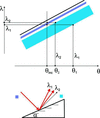Figure 1 Schematic DuMond diagram for asymmetric diffraction. A polychromatic pencil beam impinging with angle θinc is, after diffraction, sagittally deviated and spread at the same time. The spread is delimited by angles θ1 and θ2. The deviation and spread are not shown to scale. The figure shows the case when the angular acceptance (i.e. the Darwin width for an incident beam) is smaller than that for a symmetric diffraction. The asymmetry angle α is negative here.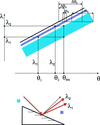Figure 2 Schematic DuMond diagram for asymmetric diffraction with positive α.

This may be easily seen from the schematic DuMond diagram (DuMond, 1937) shown in Fig. 1. At this orientation of the surface with respect to the impinging beam the Darwin width for the impinging beam is narrow and that for the diffracted beam is broad. This is depicted in the DuMond diagram by two bands: a narrow (violet) one for the impinging radiation and a broad (blue) one for the diffracted radiation. If the parallel polychromatic beam forms the angle θinc with the diffracting planes, then the diffracted radiation is spread between angles θ1 and θ2.

For a positive α the situation is shown in Fig. 2. The DuMond diagram is the same except that the blue band corresponds to the incident radiation and the violet band to the diffracted radiation. Note that in this case the wavelength grows with θ within the spread whereas in the previous case (α < 0) the wavelength decreases with θ. The geometry shown in Fig. 2extends the acceptance Δλ whereas the geometry shown in Fig. 1reduces it. The effect of meridional deviation of the diffracted beam was utilized in the study of meridional focusing on a transversal groove fabricated into a crystal surface (Hrdý & Hrdá, 2000; Hrdý et al., 2001).

For the following explanation it is necessary to determine the angles θ1 and θ2. This may be done using Fig. 2. Obviously it holds that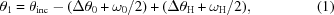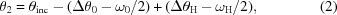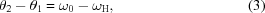where ω0 is the width of the Darwin curve for the incident radiation and Δθ0 is the deviation of its center from the Bragg angle. The meaning of ωH and ΔθH is the same for diffracted radiation.

### 3. Double-crystal asymmetric monochromator

In this section we will suppose that two asymmetric crystals with the same α are set in a non-dispersive arrangement according to Fig. 3. The two limiting beams diffracted from the first crystal are impinging on different places of the surface of the second crystal and are further diffracted under the angle θinc. It is obvious that their pathlengths become different and the original pencil beam has a width z after diffraction. The relations between the parameters are as follows.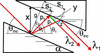Figure 3 Asymmetric double-crystal monochromator.

For grazing incidence (α > 0),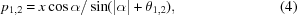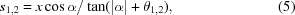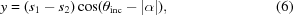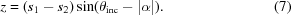The path difference Pd is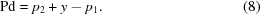For grazing emergence (α < 0),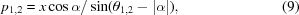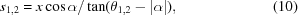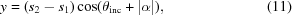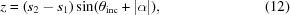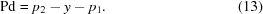The beam (θ2, λ2) obviously propagates a longer path. Note that λ2 > λ1. The front end of a positively chirped FEL pulse has a longer wavelength than the back end. This means that the front end of the pulse propagates a longer distance and thus will be retarded. If the pulse length is the same as the path difference acquired (gained) in the monochromator, then the pulse length will be substantially shortened. The necessary condition is that the divergence of the FEL pulse is much smaller than the angular acceptance of the crystal monochromator (this condition is usually fulfilled) (LCLS, 2002; SwissFEL, 2011; EuXFEL, 2007).

For an effective pulse compression it is necessary to:

(i) fit the Δλ generated by the chirping with the wavelength acceptance of the crystal;

(ii) fit the pulse length with the path difference generated in the monochromator.

The condition (i) may be fulfilled by choosing suitable α. The condition (ii) may be fulfilled by changing the mutual distance x of the two crystals.

### 4. Example

Let us suppose that the polychromatic X-ray pulse is impinging on an asymmetric Si(111) double-crystal monochromator under the angle θinc = 14°. The wavelength of the diffracted radiation is 0.1516 nm. Let the mutual distance x of the two crystals be 100 mm.

Table 1shows the values of the path difference Pd and transversal broadening z due to diffraction on both crystals for various α. t is the pulse duration which corresponds to the path difference and which may be compressed by the monochromator. The calculations are performed for x = 100 mm.

 Table 1Values of path difference Pd, transversal dimension of diffracted beam z and pulse length t which may be compressed for various asymmetry angles α
 This calculation was performed for a 100 mm mutual distance of the two crystals.
α (°)
−12 −10 +10 +12
Pd (mm) 1.008 0.132 0.004 0.007
z (mm) 4.39 0.64 0.003 0.002
t (fs) 3326.4 434 12.8 21.38
Δλ (nm) 0.00073 0.000792 0.004616 0.00916

### 5. Inclined monochromator

The inclined crystal monochromator is based on inclined diffraction (Fig. 4). In this case the plane of diffraction (i.e. the plane determined by the incident beam and the diffraction vector) is perpendicular to the plane determined by the diffraction vector and the normal to the surface. The inclined diffraction is described for example by Hrdý (1998, 2001) and Hrdý & Siddons (1999). From the theory described therein it follows that the impinging monochromatic and divergent beam is, after diffraction, sagittally deviated and sagittally spread at the same time. This is due to a boundary condition that leads to a non-coplanar diffraction. The ratio of the sagittal angular spread to the average sagittal deviation δ is the same as the ratio of the Darwin width to the deviation of the center of the Darwin curve from the exact Bragg angle θB. This may be utilized to construct the generalized DuMond diagram (Fig. 5). This is different from the classical DuMond diagram (DuMond, 1937) in that the sagittal deviation δ is plotted on the vertical axis in the direction of negative λ.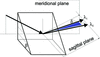Figure 4 Inclined diffraction. A polychromatic pencil beam is, after diffraction, sagittally deviated by an angle δ and spread at the same time. The deviation and spread are not shown to scale.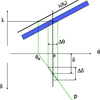Figure 5 Generalized DuMond diagram for inclined diffraction and monochromatic divergent impinging beam.

The straight line p originating at θB is oriented so that the projection of Δθ onto p creates the sagittal spread Δδ. So far we have supposed that the impinging beam is a divergent monochromatic pencil beam. Let us now suppose that the impinging beam is a parallel polychromatic pencil beam. The situation is shown in Fig. 6. The angle between the impinging beam and diffracting planes is θ. The vertical line passing through θ on the horizontal axis intersects the diffracting band and it shows that the wavelength interval Δλ from λ2 to λ1 is diffracted. Each λ from this interval has a different Bragg angle and thus also different line p. Fig. 6shows a sagittal spread for each λ if the beam had sufficient divergence. As we supposed that the beam is parallel, there is a distribution of λ within δ which is determined from the intersections of the vertical line passing through θ with lines p for each wavelength. From this relatively simple diagram it follows that there is a linear relationship between the wavelength and the angular deviation of the outgoing beams. This may be supported by a more detailed study based on dispersion surfaces in reciprocal space.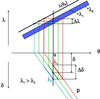Figure 6 Generalized DuMond diagram for inclined diffraction and polychromatic parallel pencil beam.

From this it may be concluded that if the impinging beam is a polychromatic parallel pencil beam then the diffracted radiation is slightly deviated and it has a dispersive spread as schematically shown in Fig. 4. For an average deviation δ and diffraction on a Si crystal it holds that (Hrdý, 1998)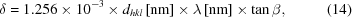where β is the inclination angle, i.e. the angle between the surface and the diffracting crystallographic planes. It is important to point out that the wavelength grows within the spread (fan) in the direction from λ2 to λ1. (Such a dispersive spread also exists in the meridional direction if the parallel polychromatic pencil beam is diffracted from an asymmetric crystal, as was shown above.) The model described above is valid for a practically feasible β, i.e. not close to 90°.

The inclined monochromator is composed of two crystals with inclined diffraction set to the parallel (non-dispersive) position (Fig. 7).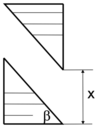Figure 7 Double-crystal inclined monochromator with an inclination angle β and the mutual distance of the two crystals defined as x.

It is obvious that the beams with different λ diffracted from the first inclined crystal are diffracted from different points on the surface of the second crystal. These beams are further diffracted in the same direction as the direction of the beam impinging on the first crystal. The situation is shown in Fig. 8. The beam diffracted from the inclined monochromator has now increased the transversal dimension and beams with different λ have different pathlengths. The pathlength difference Pd of the limiting beams may be expressed as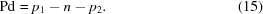p1, n and p2 may be calculated from the following relations,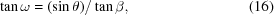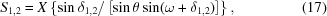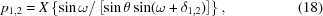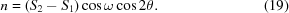The beam corresponding to δ1 has obviously a longer pathlength and longer wavelength and thus any pulse of wavelength λ1 will be slightly retarded compared with a pulse of wavelength λ2.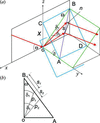Figure 8 (a) The geometry of beams diffracted from the double-crystal inclined monochromator. The diffracting crystallographic planes are horizontal (x,y plane). The impinging beam is a parallel polychromatic pencil beam. The plane of diffraction is vertical (x,z plane). The surfaces of the crystals are shown in blue. Only two limiting beams of the diffracted fan are shown. (b) A detail of (a).

This effect may be utilized for pulse compression if the FEL pulse is positively chirped. Obviously, the divergence of the pulse must be much smaller than the Darwin width of the crystal.

### 6. Example

The following estimation is made for the inclined double-crystal monochromator with Si single crystals, (111) diffraction and λ = 0.154 nm. The inclination angle β = 45°. The relative acceptance of the monochromator is Δλ/λ = 1.8 × 10−4 (the same as in the case of symmetric diffraction). The average sagittal deviation is δ = 6.06 × 10−5 rad = 12.5″. The limiting beams of the sagittal fan have the wavelengths λ1 = 0.1540138 nm (deviation of 6″) and λ2 = 0.1539862 nm (deviation of 18″). The path difference of the corresponding beams when diffracted from the second crystal is 1.13 × 10−4X [mm], where X is the vertical distance of crystals (see Fig. 7).

For example, if X = 100 mm then the pathlengths difference of the limiting beams is 0.0113 mm, which is the length of a 38 fs pulse. The divergence of existing or proposed FEL pulses is often much smaller than the Darwin width for the discussed case (LCLS, 2002; SwissFEL, 2011; EuXFEL, 2007). This means that 38 fs pulses, positively chirped in the Δλ interval, may be significantly shortened by the monochromator.

The pathlengths difference may be adjusted by changing X or β. The acceptance Δλ of the monochromator may be adjusted by introducing a certain degree of asymmetry.

The pulse compression is at the cost of the increase of the beam diameter by about 0.05 mm for X = 100 mm.

An example of how one can adjust the pulse compression using different X and β values is the following. Using an energy of E = 8.05 keV (QB = 14.21°), X = 200 mm and β = 25°, we receive a pulse length of 17.27 fs. Shortening the mutual distance of the two inclined crystals to X = 5 mm, we receive a pulse length of only 0.43 fs! A proper choice of the two parameters can provide attosecond pulse lengths.

### 7. Conclusion

We have shown that double-crystal asymmetric or inclined monochromators may be used for XFEL pulse compression. As compared with the inclined monochromator, the wavelength acceptance of the asymmetric monochromator may be set by choosing a suitable α. On the other hand, if θinc is much higher than α then the monochromator tends to behave like a symmetric crystal monochromator, for which Pd = 0. This means that the tunability of the asymmetric monochromator is limited. It would be ideal if the relative spike bandwidth Δλ/λ of a FEL is smaller than the relative wavelength acceptance of crystals. For example, the SASE spike has a bandwidth of Δλ/λ = 5 × 10−4 (Pellegrini, 2000). The simulation made by Wu et al. (2010) shows that a FWHM bandwidth of even 10−5 is possible for hard X-rays. The relative acceptance of the inclined monochromator (the same as that of the symmetric monochromator) for Si(111) is 1.8 × 10−4 and for Ge(111) it is 4.5 × 10−4. Crystals used in soft X-ray spectroscopy have even higher acceptance but are not as perfect as Si or Ge. Obviously, for a better efficiency (in terms of pulse compression and bandwidth acceptance) of the inclined monochromator, seeding is necessary. Fortunately, the acceptance may be increased by introducing a certain degree of asymmetry.

As compared with multilayers, the structure of Si crystals is much better defined. The use of gratings (Pellegrini, 2000) requires a very large mutual distance of the gratings. Whilst the wavelength acceptance in the case of inclined crystals is limited, the grating may accept a broader range of wavelengths. On the other hand, the diffraction efficiency of crystals (at least Si or Ge) is higher.

As follows from the above, the diffracted beam from the monochromator is almost parallel, it has increased transversal dimension and the corresponding pulse is very short. Such a pulse may be further focused (at least theoretically) by a parabolic mirror without influencing the pathlength difference gained in the monochromator. Other issues which should be investigated in the future are the possible impact of the crystal surface quality on the parameters of the diffracted pulse and the possible local change of structure factor caused by the impinging FEL pulse.

The average power of the XFEL will not introduce heat load problems; even more, the inclined or asymmetric geometry decreases the impinging radiation power density. Radiation damage through laser ablation can occur but this is out of the scope of this work.

In this work we did not investigate the role of extinction length in the crystal on the path difference. The effect of extinction in asymmetric crystal reduces slightly the path difference but its influence is rather small. This effect may be compensated by changing the mutual distance of the crystals.

The above explanation is based on a parallel chirped FEL beam whose wavelength changes linearly along the pulse length. In this ideal case the pulse length may be theoretically compressed to zero. However, the real beam is slightly divergent and the wavelength undergoes a scattering which overlaps the linear course. Let us suppose that these effects are small compared with the angular and wavelength acceptance of a crystal, for example n times. In this case we can very roughly estimate that the pulse length will be shortened also n times. Taking into account the parameters of some existing or proposed FELs and parameters of Ge or Si crystals, we can estimate that the pulse compression to 5–10% of the original pulse length should be easily reached (at least for an asymmetric monochromator). For an exact evaluation of the limits of the method a close cooperation with FEL experts, which would include the experiments, is necessary.

### Acknowledgements

This work was partially financed by MPO Czech Republic, grant No. MPO FR-TI1/412.

### ReferencesJOURNAL OFSYNCHROTRONRADIATION